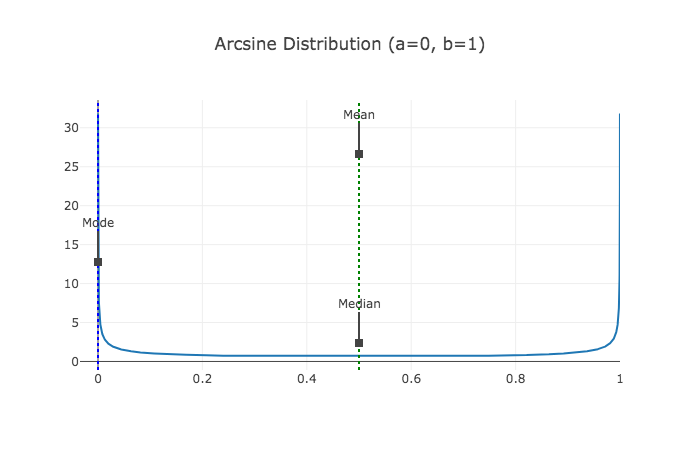## Arcsine Distribution

The PDF is

$$\frac{1}{\pi\sqrt{x(1-x)}}$$

for $x\in [0,1]$.

It can also be generalized to

$$\frac{1}{\pi\sqrt{(x-1)(b-x)}}$$

for $x\in [a,b]$.

## VisualizePublished: by ;

Lei Ma (2020). 'Arcsine Distribution', Datumorphism, 03 April. Available at: https://datumorphism.leima.is/cards/statistics/distributions/arcsine/.

Table of Contents

Current Ref:

• cards/statistics/distributions/arcsine.md